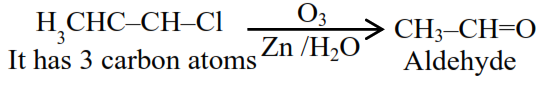# A chloro compound "A".Question:

A chloro compound "A".

(i) forms aldehydes on ozonolysis followed by the hydrolysis.

(ii) when vaporized completely $1.53 \mathrm{~g}$ of A, gives $448 \mathrm{~mL}$ of vapour at STP.

The number of carbon atoms in a molecule of compound $\mathbf{A}$ is

Solution:

$448 \mathrm{ml}$ of $\mathrm{A} \Rightarrow 1.53 \mathrm{gm} \mathrm{A}$

$22400 \mathrm{ml}$ of $\mathrm{A} \Rightarrow \frac{1.53}{445} \times 22400 \mathrm{gm} \mathrm{A}=7650$$\& \mathrm{~mm}$ is $36+5+35.5=76.5$

26 August 2021, Time 3PM to 6PM (Shift 2)